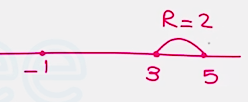Need Help?

Subscribe to Differential Equation

###### \${selected_topic_name}
• Notes

Find a lower bound for the radius of convergence of $\left(x^{2}-2 x-3\right) y^{\prime \prime}+x y^{\prime}+4 y=0$ about $x 0=\{5,-5,0\}$

$\left(x^{2}-2 x-3\right) y^{\prime \prime}+x y^{\prime}+4 y=0$

$p(x)=x^{2}-2 x-3=0 \rightarrow(x-3)(x+1)=0$

$x=3, x=-1$

$x{0}=5$